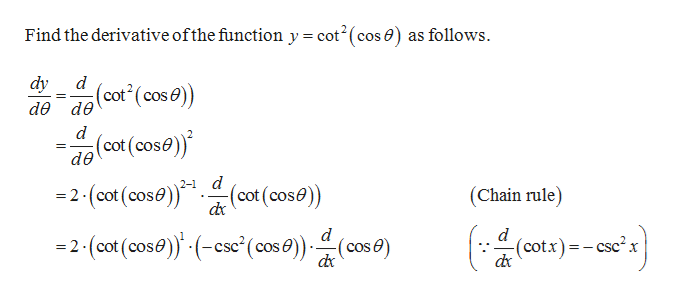y = cot2(cos(θ))

Question

y = cot2(cos(θ))

Step 1help_outlineImage TranscriptioncloseFind the derivative ofthe function y = cot2(cos ) as follows dy d de dө (cot (cose) d (cot (cose) de d =2-(cot (cose)(cot(cose) 2- (Chain rule) d d (cotx)-csc2x =2-(cot (cose) (cse'(cos e))(cos 6e) fullscreen

Want to see the full answer?

See Solution

Want to see this answer and more?

Our solutions are written by experts, many with advanced degrees, and available 24/7

See Solution
Tagged in

Derivative